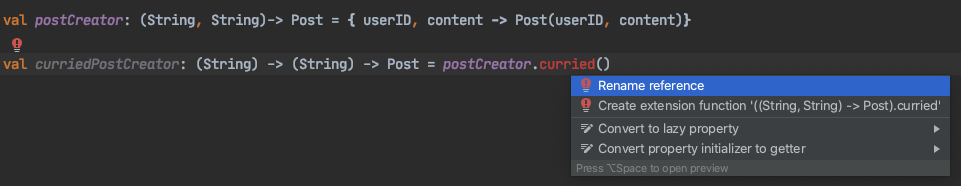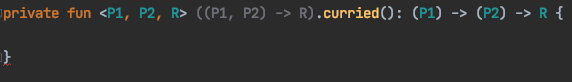#DAY 19
0
Software Development

# Simple sample

``````class Post(val userID: String, val content: String)
``````

``````val postCreator: (String, String)-> Post = { userID, content -> Post(userID, content)}
``````

``````// (String) -> (String) -> Post 跟 (String) -> ( (String) -> Post ) 是一樣的
val curriedPostCreator: (String) -> (String) -> Post = { userId ->
{ content ->
Post(userId, content)
}
}
``````

``````val postCreator: (String, String)-> Post = { userID, content -> Post(userID, content)}
val curriedPostCreator: (String) -> (String) -> Post = postCreator.curried()
````````````fun <P1, P2, R> ((P1, P2) -> R).curried(): (P1) -> (P2) -> R {
return { p1: P1 ->
{ p2: P2 ->
this(p1, p2)
}
}
}
``````

# Usecase

``````val colorWhite = ContextCompat.getColor(context, R.color.white)
val colorPrimary = ContextCompat.getColor(context, R.color.colorPrimary)
val colorPrimaryDark = ContextCompat.getColor(context, R.color.colorPrimaryDark)
``````

``````// Non-functional way
fun getColor(id: Int): Int {
return ContextCompat.getColor(this, id)
}

val colorWhite = getColor(R.color.white)
``````

``````val curriedColorGetter: (Context) -> (Int) -> Int = ContextCompat::getColor.curried()
``````

``````val simpleColorGetter: (Int) -> Int = curriedColorGetter(context)
``````

``````private fun sample(context: Context) {
val curriedColorGetter: (Context) -> (Int) -> Int = ContextCompat::getColor.curried()
val simpleColorGetter: (Int) -> Int = curriedColorGetter(context)

val colorWhite = simpleColorGetter(R.color.white)
val colorPrimary = simpleColorGetter(R.color.colorPrimary)
val colorPrimaryDark = simpleColorGetter(R.color.colorPrimaryDark)
}
``````

# Lifting

``````lifting: ((A) -> B) -> ((F[A]) -> F[B])
``````

``````(A) -> (B) -> (F[A]) -> F[B]
``````

``````((A) -> (B)) -> F[A] -> F[B]
``````

``````(A -> B, F[A]) -> F[B]
``````

``````(F[A], A -> B) -> F[B]
``````

``````fun <A, B> fmap(fa: F<A>, transform: (A) -> B): F<B>
``````

``````class F<A> {
fun <B> fmap(transform: (A) -> B): F<B> = { ...}
}
``````

# 練習時間

``````fun <P1, P2, P3, R> ((P1, P2, P3) -> R).curried(): (P1) -> (P2) -> (P3) -> R {
TODO()
}

fun <P1, P2, P3, P4, R> ((P1, P2, P3, P4) -> R).curried(): (P1) -> (P2) -> (P3) -> (P4) -> R {
TODO()
}
``````

Reference:

### 1 則留言

0

1. 先用 curry 把這個函式變成惰性求值的函式
2. 用 partial application 將已知的參數填進去
3. 用函式組合組出不同樣貌，但只差一個參數的函式。在很多語言裡有 decorator 這個概念，其實它就是個函式組合。

https://ithelp.ithome.com.tw/articles/10247503